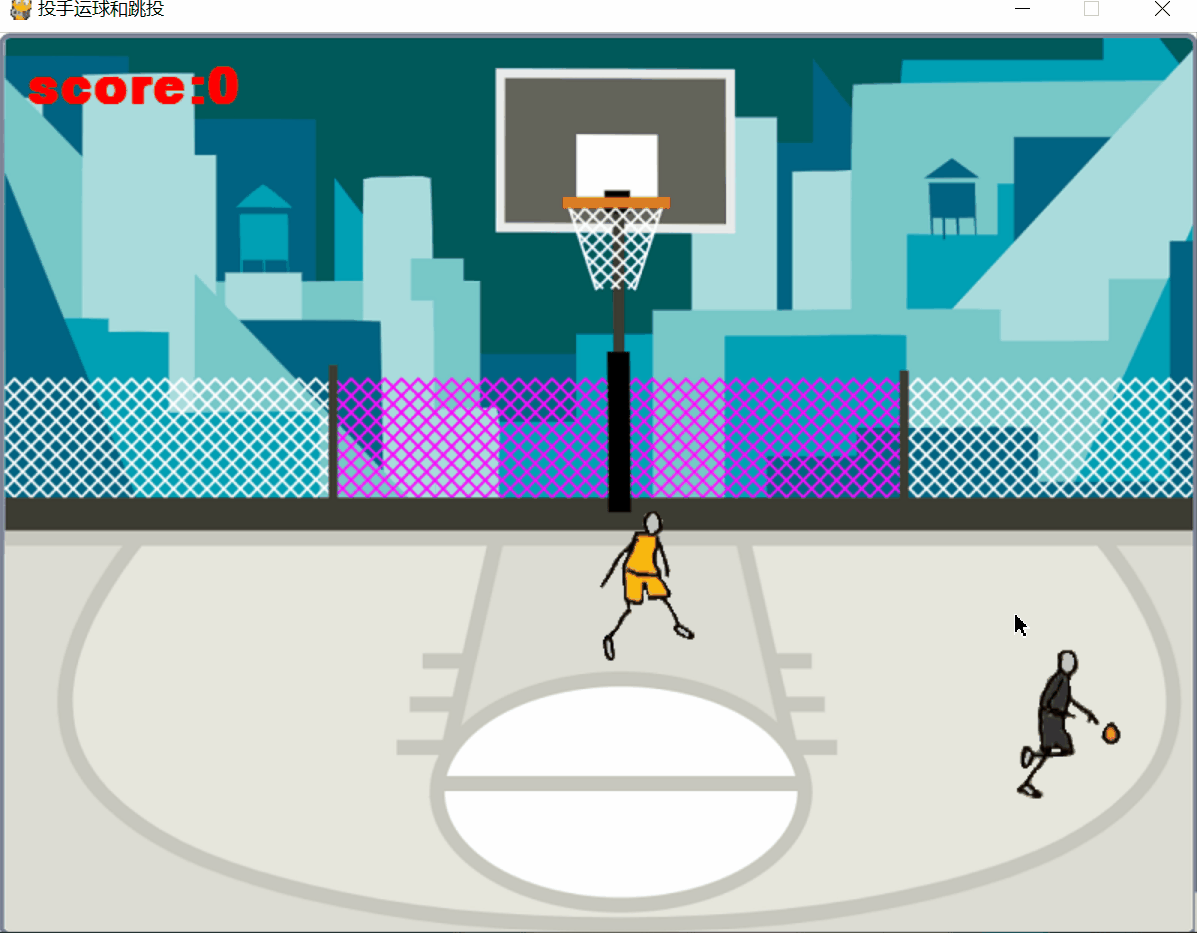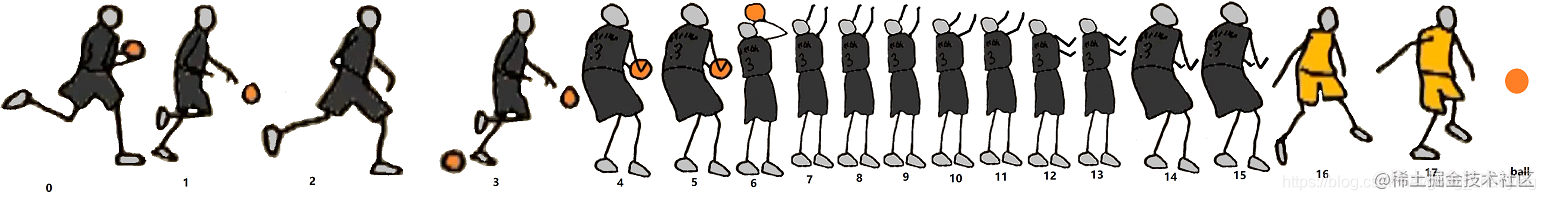# pygame编写篮球游戏-火柴人运球避开防守跳起投篮``````import pygame
import math
import random
import os
class Ball():                       #篮球类
def __init__(self,screen):      #screen是游戏主窗体，Surface类实例
self.screen=screen
r=b.get_rect()
self.p=pygame.transform.scale(b,(r.width//2,r.height//2))   #缩小图形
self.x,self.y,self.xi,self.yi=0,0,0,0#(x,y)篮球坐标,(xi,yi)是篮球两个位置间增量
self.mark=0             #此次投篮中否，=0不中，=1中
self.score=0            #投篮投中次数(得分)
def draw(self):             #主程序调用，实现篮球动画
return
dx,dy=(400-self.x),(40-self.y)  #坐标(400,530)点是球碰到篮板上的点
self.xi=dx//6                   #篮球从起始点到篮板每帧沿x轴前进的增量
self.yi=dy//6                   #篮球从起始点到篮板每帧沿y轴前进的增量
dist=math.sqrt((dx**2)+(dy**2))     #投篮点距离篮板距离
n=int(dist//100)                    #除数越小，总投中率越低
if random.randint(1,n+1)==1:        #随机数为1投中，n+1避免dist<100为0
self.mark=1                     #投中标记为1
else:
self.mark=0                     #投不中为0
self.x+=self.xi                         #篮球每帧沿x轴增加1个增量值
self.y+=self.yi                         #篮球每帧沿y轴增加1个增量值
self.x=400              #球碰到篮板的x坐标
if self.mark==1:        #投中，篮球落点y轴方向靠近篮筐
self.y=90
else:                   #投不中，篮球落点y轴方向离篮筐较远
self.y=70
else:                       #篮球下落的两个点，即第7，8帧
if self.mark==0:        #球未投中,球除下落,还沿x轴方向移动,球从篮筐两侧落下
if self.xi>=0:      #如球从左到右,最后两帧,球沿x轴方向继续从左向右移动
self.x+=30
else:
self.x-=30      #否则最后两帧，球沿x轴方向继续从右向左移动
self.y+=25              #如投中x坐标不变，即球直接下落穿过篮筐
self.screen.blit(self.p, (self.x, self.y)) #在屏幕指定位置绘制篮球
self.score+=1
class Guard():                      #防守者类
def __init__(self,screen):      ##screen是游戏主窗体，Surface类实例
self.screen=screen
self.images=[]
for n in range(2):          #将2帧图像保存到列表中
r=p.get_rect()
p = pygame.transform.scale(p, (r.width//6, r.height//6))    #调整图像的大小
self.images.append(p)
self.x,self.y=400,300           #防守运动员在窗体的初始坐标
self.PlayerX,self.PlayerY=0,0   #此时投篮手坐标
self.rect=None#调用blit绘制图形,返回rect记录图形在screen坐标和图形宽和高,用来检测碰撞
def draw(self):                     #主程序调用，实现防守者动画
if self.PlayerX-self.x<0:                   #面向投篮手
p=pygame.transform.flip(p,True,False)
dx,dy=self.PlayerX-self.x,self.PlayerY-self.y #防守者和投篮手两点沿x、y轴的差值dx和dy
dist=math.sqrt((dx**2)+(dy**2))               #计算投篮手和防守者距离
dx1,dy1=0,0                 #防守者每帧沿x轴移动dx1，沿y轴移动dy1
if dist>200:                #如距投篮者>200,返回初始点
self.x,self.y=400,300
if abs(dx)<abs(dy):     #保证abs(dy)不为0，使下句dx/dy一定不会被0除
d=abs(dx/dy)
dy1=7                #如矩形长边为7，
dx1=int((dy1*d)//1)  #dx1可能是：0，1，2，3，4，5，6，7
else:                    #保证abs(dx)不为0，使下句dy/dx一定不会被0除
d=abs(dy/dx)
dx1=7                #如矩形长边为7，
dy1=int((dx1*d)//1)  #dy1可能是：0，1，2，3，4，5，6，7
if dx<0:                     #得到dx的正负号
dx1=-dx1
if dy<0:                     #得到dy的正负号
dy1=-dy1
self.x+=dx1                  #防守者移动
self.y+=dy1#下句返回rect用来检测碰撞,其属性x,y是图形在游戏窗口坐标,width,hight是图形宽和高
self.rect=self.screen.blit(p,(self.x,self.y))   #在屏幕指定位置绘制防守者
class Player():                 #投篮手类
def __init__(self,screen):  #screen是游戏主窗体，Surface类实例
self.screen=screen
self.images=[]
for n in range(16):      #将16帧图像(包括运球和跳投图像)保存到列表中
r=p.get_rect()
p = pygame.transform.scale(p, (r.width//6, r.height//6))    #调整图像的大小
self.images.append(p)
self.x,self.y=0,0               #图像在窗体的坐标
self.mouseX,self.mouseY=0,0     #此时鼠标坐标值
self.jumpUpOrDown=-10   #按空格键后投篮手向上跳，初始值为负数。到最高点后下落，为正数
self.rect=None#调用blit绘制图形,返回rect记录图形在screen坐标和图形宽和高,用来检测碰撞
def dribble(self):          #运球动画
if self.mouseX-self.x<0:        #面向鼠标
p=pygame.transform.flip(p,True,False)
self.x,self.y=self.mouseX,self.mouseY   #投篮手坐标=鼠标坐标
if self.x<1:                            #控制投篮手必须在篮球场中
self.x=1
if self.x+90>width:
self.x=width-90
if self.y<230:
self.y=230
if self.y+120>height:
self.y=height-120
self.rect=self.screen.blit(p,(self.x,self.y)) #在指定位置绘制图形,返回rect
def jumpShot(self):                             #跳投动画
if self.x>width/2:                          #面向篮板
p=pygame.transform.flip(p,True,False)
self.screen.blit(p, (self.x, self.y))   #跳投初始位置是运球转跳投时位置
self.y+=self.jumpUpOrDown               #以后先向上(y值减少)，到最高点后下降
self.jumpUpOrDown=10
self.jumpUpOrDown=-10
pygame.init()
os.environ['SDL_VIDEO_WINDOW_POS']="%d,%d"%(200,40) #游戏窗口距左侧和顶部点数为200,40
size = width, height = 800,600                      #创建游戏窗口大小
screen = pygame.display.set_mode(size)
pygame.display.set_caption("投手运球和跳投")        #设置窗口标题

fclock = pygame.time.Clock()                        #创建控制频率的clock
fps = 4                                             #定义刷新频率
player=Player(screen)                               #投篮手类实例
ball=Ball(screen)                                   #篮球类实例
guard=Guard(screen)                                 #防守者类实例
font1 = pygame.font.SysFont('宋体', 50, True)       #创建字体
gameOver=False                                      #该次游戏是否结束，初始不结束
running = True                                      #程序是否结束，初始运行
while running:
for event in pygame.event.get():
if event.type == pygame.QUIT:               #处理退出事件
running = False                         #程序结束
if event.type == pygame.MOUSEMOTION:        #鼠标移动事件
player.mouseX,player.mouseY=event.pos   #将鼠标位置传递给投篮手用于运球
if event.type == pygame.KEYUP:              #按键后抬起事件，避免长按键不抬起
if event.key == pygame.K_SPACE:         #按空格键后抬起
if event.key == pygame.K_r and gameOver==True:     #按r键后抬起，重玩游戏
gameOver=False
ball.score=0
screen.blit(bg_img, (0, 0))             #绘制篮球场背景
surface1=font1.render('score:'+str(ball.score),True,[255,0,0])  #不能显示中文
screen.blit(surface1, (20, 20))         #显示进球数(得分)
if gameOver==True:                      #如果该次游戏结束，后边程序不再执行
fclock.tick(fps)   #fps是每秒多少帧,减去程序运行时间，为实现fps,还需延迟时间
continue
player.jumpShot()
ball.x=player.x             #球向篮板运动的起始位置
ball.y=player.y
else:                                   #如果投篮手帧号<4,投篮手正在运球
player.dribble()
ball.draw()                             #篮球动画
guard.PlayerX,guard.PlayerY=player.x,player.y   #将投篮手位置传递给防守者